# Venn Diagrams

## What are Venn diagrams?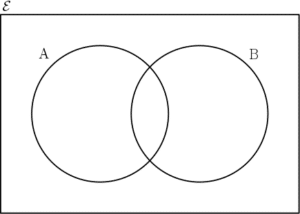A Venn diagram, named after John Venn in the 19th century, provide a convenient way to represent a sample space. Click here to remind yourself of what a sample space is.

A Venn diagram is a rectangle representing the whole space and circles inside representing various subspaces.

For example, A could represent the event that a person had blue eyes. Event B could be that person have brown hair.

## Events on a Venn diagram

### Complement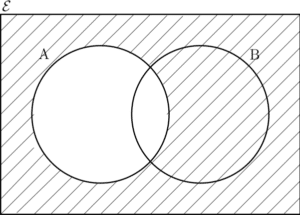This Venn diagram shows the complement of A. The part shaded with diagonal lines is everything that is not in A and we call this the complement. Note the spelling – it doesn’t say ‘compliment’ as charming as event A might be. See Example 1. The complement of A is written as:

$A^C$ or $A’$

### UnionIn this Venn diagram we see the union of events A and B. This means that the shaded part represents all outcomes where either event A or event B has occurred. See Example 2. The union of A and B is written as:

$A\cup B$

### Intersection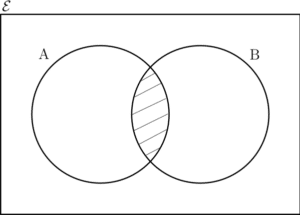Alternatively to the union, there is also the intersection of two events. The intersection represents the set of all outcomes where BOTH events A and B have occurred. See Example 3. The intersection of A and B is written as:

$A\cap B$

### Combinations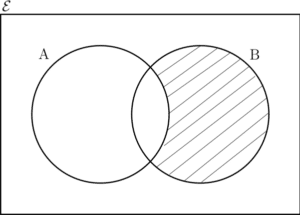It follows from the three previous definitions that it is possible to combine complement, union or intersection to get particular areas of a Venn diagram. See Example 4. The above combination is written as:

$A’\cap B$

## Venn Diagram Examples

Let event A be an even result when a dice is rolled. Additionally, let event B be the even that the result is greater than 2. The probability of not A is getting an odd result.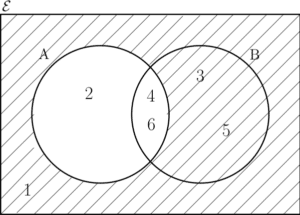Odd results are 1,3 or 5 (shaded part) and so in this case $P(A’)=3/6=1/2$.

Again, let events A and B be an even result and a result greater than 2 respectively when a dice is rolled. The probability of A or B occuring includes all of the following results: 2,3,4,5 or 6.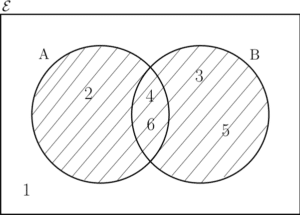It follows that$(A\cup B)=5/6$.

If A is an even result when a dice is rolled and B is a result is greater than 2, the only times you would get both are if you roll a 4 or a 6.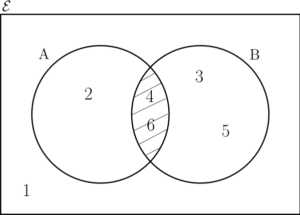It follows that the probability of the intersection is $P(A\cap B)=2/6=1/3$.

Consider again that A is an even result and B is a result greater than 2 when a dice is rolled. Results that require A not happening, i.e. an odd result, as well as B occurring includes only 3 and 5.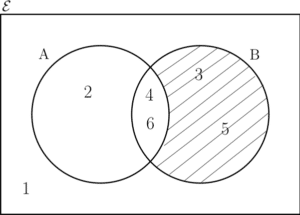Finally, in this case $P(A’\cap B)=2/6=1/3$.

A card is chosen at random from a standard deck of 52 playing cards. Let K be the event that the card is a King. Let H be the event that the card is a heart. Find:

1. $P(K\cap H)$
2. $P(H’)$
3. $P(K\cup H)$
4. $P(K’\cap H’)$

A school has 204 students. 85 of them have chosen to study Maths, 56 of them have chosen to study French and 68 have chosen to study History. It turns that 35 study both French and Maths, 23 study both Maths and History and 27 study both History and French. 7 study all three subjects.

1. Draw a Venn diagram to represent this information.
2. Find the probability that a student does not study Maths.
3. Find the probability that a student does none of these three subjects.

…coming soon.